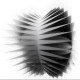Sketches of radiolarianfrom The Voyage of H.M.S. ‘Challenger’ circa 1858. ‘Radiolarians condense their structure according to the flow of forces by controlling the diameter of the individual bubbles intersecting the global sphere in the radiolarian. Bubble clusters with a high amount of small diameter bubbles have a large amount of membrane surface, which finally results in a denser skeleton structure. The density of the skeletal structure reflects the amount of stress that can be carried within the structure. In this way radiolarians optimize the geometry of the grid of the skeletal structure according to the magnitude and the direction of the applied loads. This principle can be used for an optimization algorithm. As the first step the surface of the structure, which is unloaded, is divided into equal sized spheres in their closes packing (with six spheres round each sphere in a hexagonal grid). Each sphere is in a membrane stress situation like a soap bubble, for which the Laplace-Young equation (Isenbergholds:p=2*q/r(p= internal pressure, q=stress, r=radius). By assuming the stress in each bubble as constant throughout the optimization procedure the internal pressure in the bubbles will represent as a measure of the internal stress in the structure. When the structure is loaded the internal stress in the structure will not be equal anymore in all places. Because we take the membrane stress as a constant with the Laplace-Young equation we can see that radius of each bubble will change according to its pressure. This will result in an optimized configuration of bubbles, in which the stress in each bubble is the same.

For the way to solve this optimization process as linear optimization formulation has been used as is applied in the “Thrust Network Analysis”, in this way a more holistic way of optimization is achieved without an endless process of iterations with vary little variations in the numerous solutions. Ways of extending the proposed optimization routine is to take into account the deformations of the spheres during optimization without volume and pressure change or with volume and pressure change by using Boyle’s Law (P0V0 = P1V1(P=pressure, V=Volume, 0=before change, 1=after change). The benefit of the first extension is that the bubbles do not have to remain as spheres during the optimization, which gives an added design variable. The second extension would also give the added possibility of, besides form change, varying the membrane stresses and allowing the air inflated cushions (bubbles) to structurally behave differently.’1

1 – Borgart, Andrew. Stach, Edgar. Computational structural form finding and optimization of pneumatic structures
Stress
Pressure
Volume

Categories: Biomimetics#### Author:jonbailey

studying: architecture design